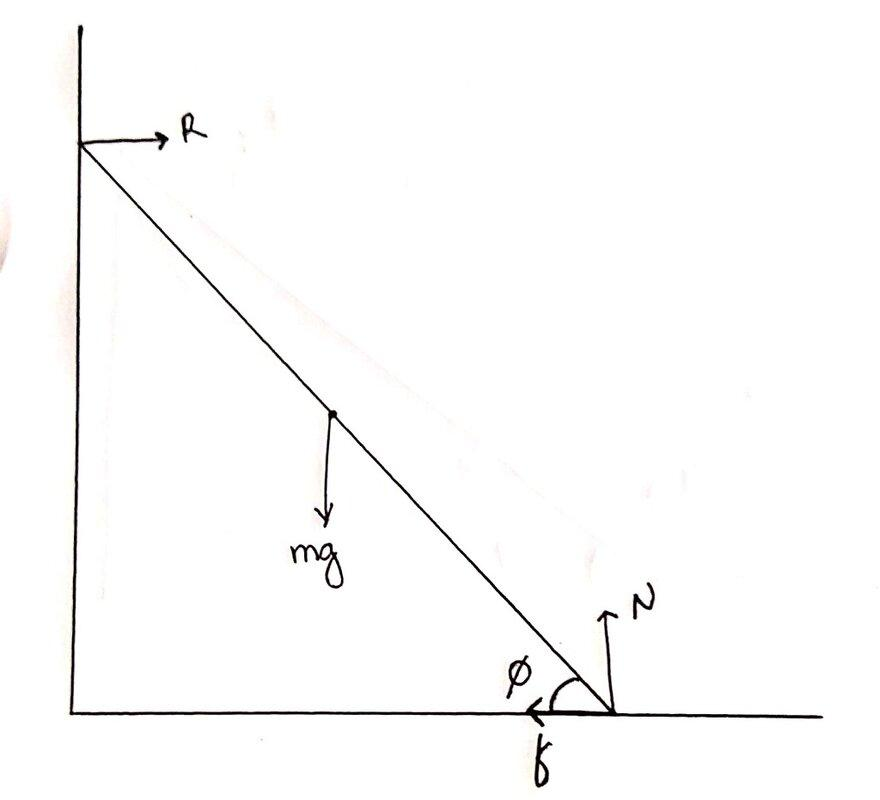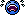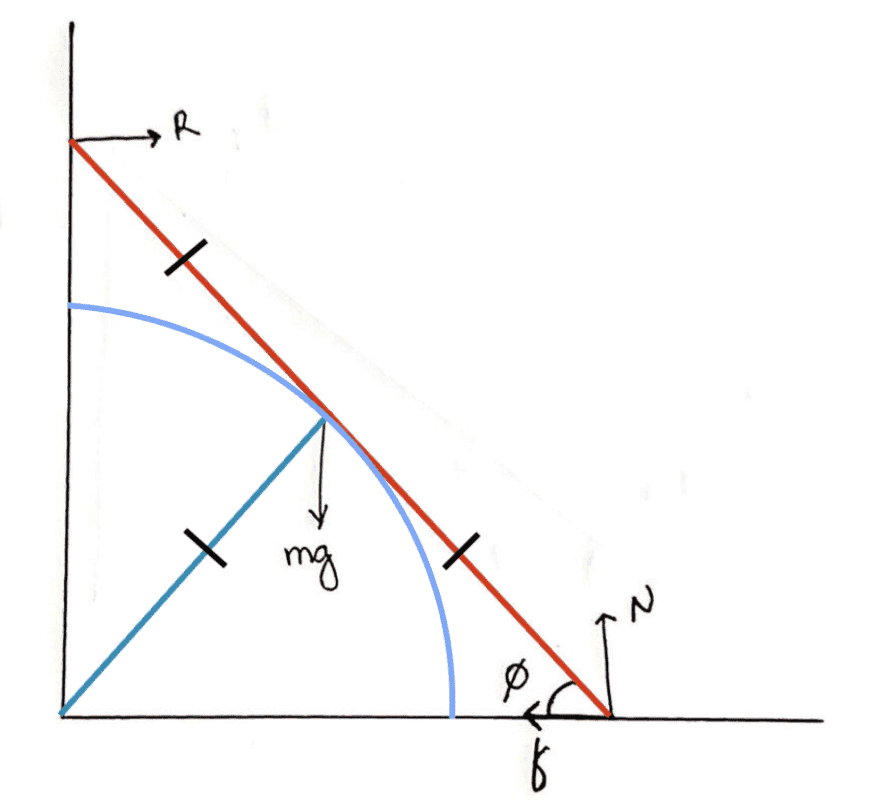# The leaning ladder problem using Newtonian mechanics

• Hamiltonian
In summary, the conversation discusses a problem involving a ladder sliding down a wall with friction and normal reactions being the only forces acting on it. The conversation goes on to explore different methods for solving the problem, such as using equations of motion and the Euler-Lagrange equation. However, it is concluded that the most effective approach would be to consider the motion of the ladder's center of mass, which traces out a circular trajectory of radius L/2. The person asking the question expresses difficulty in solving the problem, but their teacher considers it to be a trivial one.

#### Hamiltonian

Homework Statement
A uniform rod of mass M and length is placed against a smooth wall. the coefficient of kinetic friction between the floor and the ladder is cot(phi)/4
where phi the initial angle of inclination of the ladder. Find the time taken by the ladder to slip and become horizontal.
Relevant Equations
T = I##\alpha##
F = ma$$R - f = m\ddot x$$ $$N - mg = m\ddot y$$
were N and R are the normal reactions from the smooth wall and rough ground.
and f is the friction provided by the ground.
$$f = \mu N = cot(\phi)N/4$$

i tried to formulate a constraint relation between ##\ddot x## and ##\ddot y## so that I could solve the above equations
since $$x^2 + y^2 = L^2$$
$$x \frac {dx} {dt} = y \frac {dy} {dt}$$
$$\frac {dx}{dt} = tan(\theta) \frac {dy} {dt}$$
$$\ddot x = \ddot y tan(\theta) + \dot y \dot \theta sec^2(\theta)$$
the problem here is the ##\dot y##!
also ##\theta## is the angle made by the ladder at some time during its motion(##\theta## changes with time)

Taking the torques about the center of mass of the rod gives me
$$(Ncos(\theta) - fsin(\theta) - Rsin(\theta))L/2 = I \frac {d(\omega)} {dt}$$
$$\frac{L}{2I}\int_0^t t\,dt = \int_\phi^0 \frac {d\theta} {(Ncos(\theta) - fsin(\theta) - Rsin(\theta)}$$
here I end up with an integral that I am unable to solve!can someone tell me if I am doing something conceptually wrong here or if I have made some major errors in my process?
also would there be an easier way to solve this question as my teacher called it "trivial" and I can't seem to get my head around it.
thanks:)

Last edited by a moderator:
What exactly do ##x## and ##y## represent in your equations? It looks like they are the distances of the two ends of the ladder from the corner. If that's the case, you cannot write the Newton's second law equations as you have. Consider instead the motion of the center of mass. Can you imagine what kind of path it describes as the ladder slides?

•etotheipi
kuruman said:
What exactly do ##x## and ##y## represent in your equations? It looks like they are the distances of the two ends of the ladder from the corner. If that's the case, you cannot write the Newton's second law equations as you have. Consider instead the motion of the center of mass. Can you imagine what kind of path it describes as the ladder slides?
yes, they are the distances of the ends of the ladder from the corner.
ok I think I understand the mistake I have made here if let's say ##x## and ##y## are the coordinates of the center of mass then there would be only a slight change in the equations
$$x^2 + y^2 = (L/2)^2$$

but again this will become
$$x\frac {dx}{dt} = y\frac{dy}{dt}$$
and hence the other equations will remain the same.
also the torque equations?

This equation$$x\frac {dx}{dt} = y\frac{dy}{dt}$$ isn't much help. You have three equations and three unknowns, ##N##,##R## and ##\theta##. You need to use the two force equations to eliminate ##R## and ##N## from the torque equation to get the equation of motion involving ##\theta## only and see what it looks like. It's probably something horrible that cannot be solved analytically.

Your other option would be to use the work energy theorem to get an expression for ##\frac{d\theta}{dt}## and see if you can integrate that. Finally, I am not sure that the rod maintains contact with the wall at all times. You may need to investigate that.

•Hamiltonian
kuruman said:
Finally, I am not sure that the rod maintains contact with the wall at all times. You may need to investigate that.
there is no external force pulling the rod to the right its only gravity friction and the normal reactions hence I concluded that the rod will always remain in contact also the rod does not have a significant amount of width to it.
would it be easier to get equations of motion by using the Euler Lagrange equation(i have not used it per se to solve mechanics problems yet and do not know how to apply them properly hence I ask)

I don't think it be easier to use Euler-Lagrange for this especially since friction is involved and you do not know how to do it. I would go with the way I understand.

Construct an imaginary line segment between the corner and the centre of mass of the rod. As the ladder falls, assuming it always remains in contact with the two walls, the geometry of the problem looks like this:Notice that there are two isosceles triangles present in the figure; consequently, the line segment from the corner to the centre of mass is of constant length ##L/2##. You deduce that the centre of mass traces out a circular trajectory of radius ##L/2##.

••Lnewqban and Hamiltonian
Hamiltonian299792458 said:
also would there be an easier way to solve this question as my teacher called it "trivial" and I can't seem to get my head around it.
thanks:)

It says on your profile you are in high school. This isn't a high school problem, let alone a trivial one. What's going on here?

•etotheipi
PeroK said:
It says on your profile you are in high school. This isn't a high school problem, let alone a trivial one. What's going on here?
I am in 11th grade. This is indeed a problem given to me by my teacher and he did refer to it as trivial so...?
also JEE adv syllabus is very similar to first year of college syllabus.
we are obviously not taught about lagrangians in high school i just asked about that out of curiosityI think this problem would not be out of place in a Physics olympiad. It certainly wouldn't be out of reach for the IPhO. But I agree, it's not trivial.

Hamiltonian299792458 said:
I am in 11th grade. This is indeed a problem given to me by my teacher and he did refer to it as trivial so...?
also JEE adv syllabus is very similar to first year of college syllabus.
we are obviously not taught about lagrangians in high school i just asked about that out of curiosityI haven't looked at this particular problem but in general whenever things get complicated solving for a function of time is usually not possible. There might be a trick based on the strange coefficient of friction, but I would be skeptical.

PeroK said:
I haven't looked at this particular problem but in general whenever things get complicated solving for a function of time is usually not possible. There might be a trick based on the strange coefficient of friction, but I would be skeptical.
the coefficient of friction is exactly half of what would be needed to keep the ladder in static equilibrium at an angle of inclination ##\phi##
but I am not too sure as to how this info is of any use

•ehild
etotheipi said:
I think this problem would not be out of place in a Physics olympiad. It certainly wouldn't be out of reach for the IPhO. But I agree, it's not trivial.
Have you solved it?

PeroK said:
Have you solved it?

Not today, but I remembered this question from a maths homework. I recall the key was to determine the trajectory of the centre of mass, which I gave in #7. I hope I'm not missing something, or otherwise I'm going to look really stupid...•PeroK
You will find a derivation of the relevant equations here. It is a bit more general in that there is friction at the wall contact (same ##\mu##) as well. It is not a trivial problem. At least I would love to see the teacher's solution.

•BvU, Hamiltonian and PeroK
etotheipi said:
assuming it always remains in contact with the two walls
That's what makes this problem interesting, it can't.

•etotheipi
haruspex said:
That's what makes this problem interesting, it can't.
I am not able to see why it would lose contact with the wall? there is no force towards the right other than ##R##(normal reaction from wall) also the rod is of negligible thickness.
so why exactly should it loose contact?

Hamiltonian299792458 said:
I am not able to see why it would lose contact with the wall? there is no force towards the right other than ##R##(normal reaction from wall) also the rod is of negligible thickness.
so why exactly should it loose contact?
If it were to maintain contact all the way, when it reaches the horizontal it would have zero horizontal velocity. How can that happen?

•PeroK
kuruman said:
Your other option would be to use the work-energy theorem to get an expression for ##\frac{d\theta}{dt}## and see if you can integrate that.
i have found the initial potentia lenergy of the rod as $$U = \frac {MgLsin(\phi)}{2}$$
will the final kinetic energy of the rod be $$= 1/2(m)[\dot x^2 + \dot y ^2] + 1/2 I \omega ^2$$
also will the work done by friction$$= \mu NL(1 - cos(\phi))$$

POST RETRACTED due to different interpretations of OP.

haruspex said:
If it were to maintain contact all the way, when it reaches the horizontal it would have zero horizontal velocity. How can that happen?
That's alright, the friction between ladder and floor is infinite at that point, and the horizontal speed is Zero.

friction = cot(theta)/4
theta = 0
cot(0) =

speedHORIZ = tan(theta)

Edit: The OP states in post 24 below that for calculation purposes he has defined phi as theta. The statements made in this post are changed to reflect this.
end Edit:

Last edited:
•PeroK
Hamiltonian299792458 said:
will the work done by friction
The normal force reduces as the rod slides, so the frictional force reduces too. And I don't think you can take these as being the same as for the static case at a given angle. Some of the gravity will be providing the centripetal force, reducing the normal force from the floor.

POST RETRACTED due to different interpretations of the OP

haruspex said:
How so? The normal force is headed to zero.
The problem statement defines phi as the angle between the ladder and the floor. It also defines the friction between the two as cot(theta)/4.

As the ladder falls, phi approaches Zero and the cotan of Zero is infinite, in most of the universe /4 = .

Ergo, the friction approaches infinity as the ladder approaches horizontal.

Edit: The OP states in post 24 below that for calculation purposes he has defined phi as theta. The statements made in this post are changed to reflect this.
end Edit:

Last edited:
Tom.G said:
The problem statement defines phi as the angle between the ladder and the floor. It also defines the friction between the two as cot(phi)/4.

As the ladder falls, phi approaches Zero and the cotan of Zero is infinite, in most of the universe /4 = .

Ergo, the friction approaches infinity as the ladder approaches horizontal.
##\phi## is only the initial angle of inclination it is a constant it does not approach zero! also, can the coefficient of friction between any two surfaces ever be variable?
(I defined ##\theta## as the angle that approaches zero.)

•PeroK
[/QUOTE]
Edit: The OP states in post 24 below that for calculation purposes he has redefined phi as theta. The statements made in this post remain applicable regardless of the naming of the angle between the ladder and the floor.
end Edit:
[/QUOTE]
##\mu## cannot depend on ##\theta## its a constant! the frictional force may vary with time but the coefficient of friction between the two surfaces remains the same! and if you conclude that the frictional force approaches infinity ur implying the normal reaction on the rod by the ground becomes infinity as $$f = \mu N$$ which I don't think is the case. please correct me if I am wrongTom.G said:
Edit: The OP states in post 24 below that for calculation purposes he has defined phi as theta. The statements made in this post are changed to reflect this.
end Edit:
He did no such thing. He defined theta as the angle in general position, preserving phi for its stated meaning in the problem description, namely, the initial angle.Yup. I have retracted my posts 20 and 23. I'll go away now...•PeroK
Hamiltonian299792458 said:
the coefficient of friction is exactly half of what would be needed to keep the ladder in static equilibrium at an angle of inclination ##\phi##
but I am not too sure as to how this info is of any use
Presumably, just that was the intention of the problem maker, to make the set-up static. but he/she failed to determine the correct friction coefficient .

ehild said:
Presumably, just that was the intention of the problem maker, to make the set-up static. but he/she failed to determine the correct friction coefficient .
actually the first part of the question was to determine the least value of ##\mu## to keep the rod in static equilibrium and then in the second part they intentionally gave half of the previously found answer. also, they asked us to find the time it takes the rod to become horizontal so the ##\mu## has to be lesser.